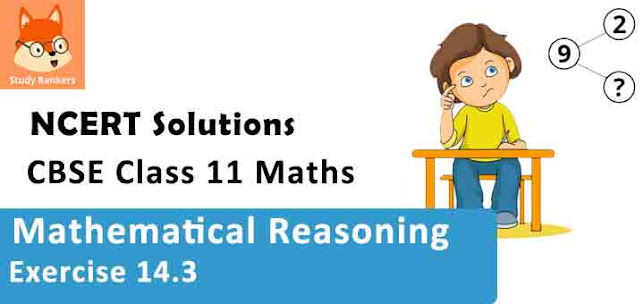# Class 11 Maths NCERT Solutions for Chapter 14 Mathematical Reasoning Exercise 14.3### Mathematical Reasoning Exercise 14.3 Solutions

1. For each of the following compound statements first identify the connecting words and then break it into component statements.
(i) All rational numbers are real and all real numbers are not complex.
(ii) Square of an integer is positive or negative.
(iii) The sand heats up quickly in the Sun and does not cool down fast at night.
(iv) x = 2 and x = 3 are the roots of the equation 3x2 – x – 10 = 0.

Solution

(i) Here, the connecting word is ‘and’.
The component statements are as follows.
p: All rational numbers are real.
q: All real numbers are not complex.

(ii) Here, the connecting word is ‘or’.
The component statements are as follows.
p: Square of an integer is positive.
q: Square of an integer is negative.

(iii) Here, the connecting word is ‘and’.
The component statements are as follows.
p: The sand heats up quickly in the sun.
q: The sand does not cool down fast at night.

(iv) Here, the connecting word is ‘and’.
The component statements are as follows.
p: x = 2 is a root of the equation 3x2 – x – 10 = 0
q: x = 3 is a root of the equation 3x2 – x – 10 = 0

2. Identify the quantifier in the following statements and write the negation of the statements.
(i) There exists a number which is equal to its square.
(ii) For every real number xx is less than x + 1.
(iii) There exists a capital for every state in India.

Solution

(i) The quantifier is “There exists”.
The negation of this statement is as follows.
There does not exist a number which is equal to its square.

(ii) The quantifier is “For every”.
The negation of this statement is as follows.
There exist a real number x such that x is not less than x + 1.

(iii) The quantifier is “There exists”.
The negation of this statement is as follows.
There exists a state in India which does not have a capital.

3. Check whether the following pair of statements is negation of each other. Give reasons for the answer.
(i) x + y = y + x is true for every real numbers x and y.
(ii) There exists real number x and y for which x + y = y + x.

Solution

The negation of statement (i) is as follows.
There exists real number x and y for which x + y ≠ y + x. This is not the same as statement (ii).
Thus, the given statements are not the negation of each other.

4. State whether the “Or” used in the following statements is “exclusive “or” inclusive. Give reasons for your answer.
(i) Sun rises or Moon sets.
(ii) To apply for a driving licence , you should have a ration card or a passport.
(iii) All integers are positive or negative.

Solution

(i) Here, “or” is exclusive because it is not possible for the Sun to rise and the moon to set together.

(ii) Here, “or” is inclusive since a person can have both a ration card and a passport to apply for a driving licence.

(iii) Here, “or” is exclusive because all integers cannot be both positive and negative.